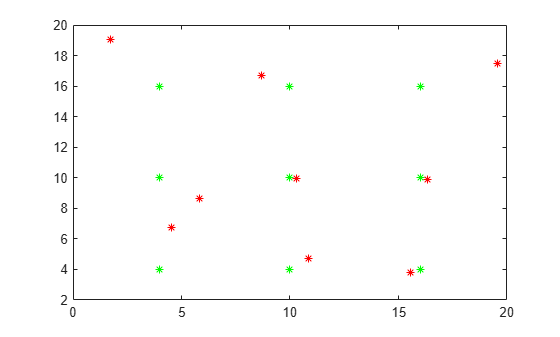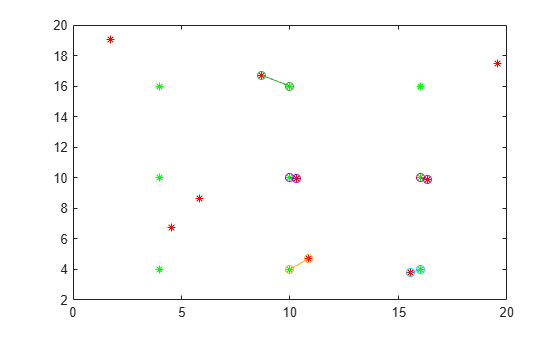# matchpairs

Solve linear assignment problem

Since R2019a

## Syntax

``M = matchpairs(Cost,costUnmatched)``
``[M,uR,uC] = matchpairs(Cost,costUnmatched)``
``[___] = matchpairs(Cost,costUnmatched,goal)``

## Description

example

````M = matchpairs(Cost,costUnmatched)` solves the linear assignment problem for the rows and columns of the matrix `Cost`. Each row is assigned to a column in such a way that the total cost is minimized. `costUnmatched` specifies the cost per row of not assigning each row, and also the cost per column of not having a row assigned to each column.```
````[M,uR,uC] = matchpairs(Cost,costUnmatched)` additionally returns indices for unmatched rows in `uR` and indices for unmatched columns in `uC`.```

example

````[___] = matchpairs(Cost,costUnmatched,goal)` specifies the goal of the optimization using any of the output argument combinations in previous syntaxes. `goal` can be `'min'` or `'max'` to produce matches that either minimize or maximize the total cost.```

## Examples

collapse all

Assign salespeople to flights such that the total cost of transportation is minimized.

A company has four salespeople who need to travel to key cities around the country. The company must book their flights, and wants to spend as little money as possible. These salespeople are based in different parts of the country, so the cost for them to fly to each city varies.

This table shows the cost for each salesperson to fly to each key city.

Each city represents a sales opportunity. If a city is missed, then the company loses out on an average revenue gain of \$2,000.

Create a cost matrix to represent the cost of each salesperson flying to each city.

```C = [600 670 960 560 900 280 970 540 310 350 950 820 325 290 600 540];```

Use `matchpairs` to assign the salespeople to the cities with minimal cost. Specify the cost of unassignment as 1000, since the cost of unassignment is counted twice if a row and a column remain unmatched.

`M = matchpairs(C,1000)`
```M = 4×2 3 1 2 2 4 3 1 4 ```

`matchpairs` calculates the least expensive way to get a salesperson to each city.

Match rows to columns when you have many more columns than rows in the cost matrix.

Create a 3-by-8 cost matrix. Since you have only three rows, `matchpairs` can produce at most three matches with the eight columns.

```rng default % for reproducibility C = randi([10 100], 3, 8)```
```C = 3×8 84 93 35 97 97 22 82 13 92 67 59 24 54 48 97 87 21 18 97 98 82 93 69 94 ```

Use `matchpairs` to match the rows and columns of the cost matrix. To get the maximum number of matches, use a large cost of unassignment (relative to the magnitude of the entries in the cost matrix). Specify three outputs to return the indices of unmatched rows and columns.

`[M,uR,uC] = matchpairs(C,1e4)`
```M = 3×2 3 2 2 4 1 8 ```
```uR = 0x1 empty double column vector ```
```uC = 5×1 1 3 5 6 7 ```

Five of the columns in `C` are not matched with any rows.

Assign taxis to routes such that the profit is maximized.

A taxi company has several ride requests from across the city. The company wants to dispatch its limited number of taxis in a way that makes the most money.

This table shows the estimated taxi fare for each of five ride requests. Only three of the five ride requests can be filled.

Create a profits matrix to represent the profits of each taxi ride.

```P = [5.7 6.3 3.1 4.8 3.5 5.8 6.4 3.3 4.7 3.2 5.7 6.3 3.2 4.9 3.4];```

Use `matchpairs` to match the taxis to the most profitable rides. Specify three outputs to return any unmatched rows and columns, and the `'max'` option to maximize the profits. Specify the cost of unassignment as zero, since the company makes no money from unfilled taxis or ride requests.

```costUnmatched = 0; [M,uR,uC] = matchpairs(P,costUnmatched,'max')```
```M = 3×2 1 1 2 2 3 4 ```
```uR = 0x1 empty double column vector ```
```uC = 2×1 3 5 ```

`matchpairs` calculates the most profitable rides to fill. The solution leaves ride requests 3 and 5 unfilled.

Calculate the total profits for the calculated solution. Since `costUnmatched` is zero, you only need to add together the profits from each match.

`TotalProfits = sum(P(sub2ind(size(P), M(:,1), M(:,2))))`
```TotalProfits = 17 ```

Use `matchpairs` to track the movement of several points by minimizing the total changes in distance.

Plot a grid of points at time $\mathit{t}=0$ in green. At time $\mathit{t}=1$, some of the points move a small amount in a random direction.

```[x,y] = meshgrid(4:6:16); x0 = x(:)'; y0 = y(:)'; plot(x0,y0,'g*') hold on rng default % for reproducibility x1 = x0 + randn(size(x0)); y1 = y0 + randn(size(y0)); plot(x1,y1,'r*')```Use `matchpairs` to match the points at $\mathit{t}=0$ with the points at $\mathit{t}=1$. To do this, first calculate a cost matrix where `C(i,j)` is the Euclidean distance from point `i` to point `j`.

```C = zeros(size(x).^2); for k = 1:length(y1) C(k,:) = vecnorm([x1(k)-x0; y1(k)-y0],2,1)'; end C```
```C = 9×9 2.8211 3.2750 9.2462 6.1243 6.3461 10.7257 11.7922 11.9089 14.7169 4.9987 2.2771 7.5752 6.2434 4.3794 8.4485 11.1792 10.2553 12.5447 15.2037 9.3130 3.7833 17.1539 12.2408 8.7988 20.7211 16.8803 14.5783 6.9004 8.6551 13.1987 1.1267 5.3446 11.3075 5.1888 7.3633 12.3901 8.6703 6.3191 8.7571 5.9455 0.3249 6.0714 8.2173 5.6816 8.3089 13.5530 8.1918 4.7464 12.7818 6.8409 1.4903 14.6652 9.9242 7.3426 11.5682 13.1257 16.8150 5.5702 8.3359 13.4144 0.4796 6.2201 12.2127 13.6699 12.3432 13.7784 8.6461 6.3438 8.8167 5.8858 0.3644 6.1337 20.6072 17.2853 15.6495 16.5444 12.1590 9.6935 13.9562 8.3006 3.8761 ```

Next, use `matchpairs` to match the rows and columns in the cost matrix. Specify the cost of unassignment as 1. With such a low cost of unassignment relative to the entries in the cost matrix, it is likely `matchpairs` will leave some points unmatched.

`M = matchpairs(C,1)`
```M = 5×2 4 4 5 5 6 6 7 7 8 8 ```

The values `M(:,2)` correspond to the original points $\left({\mathit{x}}_{0},{\mathit{y}}_{0}\right)$, while the values `M(:,1)` correspond to the moved points $\left({\mathit{x}}_{1},{\mathit{y}}_{1}\right)$.

Plot the matched pairs of points. The points that moved farther than `2*costUnmatched` away from the original point remain unmatched.

```xc = [x0(M(:,2)); x1(M(:,1))]; yc = [y0(M(:,2)); y1(M(:,1))]; plot(xc,yc,'-o')```## Input Arguments

collapse all

Cost matrix. Each entry `Cost(i,j)` specifies the cost of assigning row `i` to column `j`.

Data Types: `single` | `double`

Cost of not matching, specified as a scalar. `matchpairs` compares the value of `2*costUnmatched` to the entries in `Cost` to determine whether it is more beneficial for a row or column to remain unmatched. Use this parameter to make matches more or less likely in the algorithm. For more information, see linear assignment problem.

Example: `M = matchpairs(C,10)` specifies a cost of 10 for not matching a row or column of `C`.

Data Types: `single` | `double`

Optimization goal, specified as either `'min'` or `'max'`. The optimization goal specifies whether the total cost should be minimized or maximized.

Example: `M = matchpairs(Cost,costUnmatched,'max')` specifies that the rows and columns of `Cost` should be matched together to maximize the total cost.

## Output Arguments

collapse all

Matches, returned as a matrix. `M` is a `p`-by-`2` matrix, where `M(i,1)` and `M(i,2)` are the row and column indices of a matched pair in the cost matrix. The rows of `M` are sorted with the second column in ascending order.

• Each row and column can be matched a single time only, so each `M(i,1)` value and each `M(i,2)` value is unique.

• `M` contains `p` matches, and `p` is less than or equal to the maximum number of matches `min(size(Cost))`.

• The cost of the matches in `M` is ```sum([Cost(M(1,1),M(1,2)), Cost(M(2,1),M(2,2)), ..., Cost(M(p,1),M(p,2))])```.

Unassigned rows, returned as a column vector of indices. The entries in `uR` indicate which rows in `Cost` are unassigned. Each entry in `uR` and `uC` contributes to the total cost of the solution according to `costUnassigned`.

Unassigned columns, returned as a column vector of indices. The entries in `uC` indicate which columns in `Cost` are unassigned. Each entry in `uR` and `uC` contributes to the total cost of the solution according to `costUnassigned`.

collapse all

### Linear Assignment Problem

The linear assignment problem is a way of assigning rows to columns such that each row is assigned to a column and the total cost of the assignments is minimized (or maximized). The cost of assigning each row to each column is captured in a cost matrix. The entry `Cost(i,j)` is the cost of assigning row `i` to column `j`.

The cost of unassignment assigns a cost to any row or column that is not matched. This practice allows for minimum-cost solutions that do not assign all rows or columns. If a row and column are not matched, this increases the total cost by `2*costUnmatched`.

The total cost of a solution `M` is the sum of the cost of all matched pairs added to the cost of all unmatched pairs:

`$TC=\sum _{i=1}^{p}\mathrm{Cost}\left(M\left(i,1\right),M\left(i,2\right)\right)+\mathrm{costUnmatched}\text{\hspace{0.17em}}\cdot \text{\hspace{0.17em}}\left(m+n-2p\right)$`

In code the total cost is

```CostAssigned = sum(Cost(sub2ind(size(Cost), M(:,1), M(:,2)))); CostUnassigned = costUnmatched*(sum(size(Cost))-2*size(M,1)); TotalCost = CostAssigned + CostUnassigned;```

• `Cost` is an `m`-by-`n` matrix.

• `M` is a `p`-by-`2` matrix, where `M(i,1)` and `M(i,2)` are the row and column of a matched pair.

• `(m+n-2*p)` is the total number of unmatched rows and columns.

 Duff, I.S. and J. Koster. "On Algorithms For Permuting Large Entries to the Diagonal of a Sparse Matrix." SIAM J. Matrix Anal. & Appl. 22(4), 2001. pp 973–996.